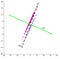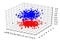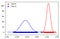# Fisher’s Linear Discriminant Analysis

It’s challenging to convert higher dimensional data to lower dimensions or visualize the data with hundreds of attributes or even more. Too many attributes lead to overfitting of data, thus results in poor prediction.

Dimensionality reduction is the best approach to deal with such data. A large number of attributes in dataset leads may lead to overfitting of datasets. There are several techniques for dimensionality reduction. The three popular dimensionality reduction techniques to identify the set of significant features and reduce dimensions of the dataset are

1) Principle Component Analysis (PCA)

2) Linear Discriminant Analysis (LDA)

3) Kernel PCA (KPCA)

In this article, we are going to look into Fisher’s Linear Discriminant Analysis from scratch.

LDA is a supervised linear transformation technique that utilizes the label information to find out informative projections. A proper linear dimensionality reduction makes our binary classification problem trivial to solve. In this classification, the dimensionality feature space is reduced to a 1-D dimension by projecting on the vector.(https://cs.nju.edu.cn/wujx/teaching/06_FLD.pdf)

In this approach, we take the shadow of points along the line or hyperplane. Through visual inspection, we can tell that it is easy to classify these examples into two classes that are positive and negative if we project points into the projection direction labeled as “FLD” (the solid green line). After the projection, the positive class examples will clump into a small range of values, as do the negative examples. However, it must be ensured that the range of projected values in different classes never overlap.

Projecting data from d-dimensions onto a line

Given a set of the n-D dimension feature vector of x1, x2, x3……, xn.

We want to form a linear combination of the components of x as:

Where w denotes the direction of the FLD vector. There can be several values of w, as shown below (for binary classification)(ref: https://cedar.buffalo.edu/~srihari/CSE555/Chap3.Part6.pdf)

As you can see, for better separation, we need to find appropriate direction w. So, to find it let define two terms (for binary classification):

Here, m1-m2 is the mean difference between the two classes. SB is called the between-class scatter matrix, and SW is the within-class scatter matrix (both are D×D in size). SW measures how scattered is the original input dataset within each class. SB measures the scatter caused by the two-class means, which measures how scattered it is between different classes.

FLD objective function is given by J as:

In other words, this optimization aims at finding a projection direction that makes the between-class scatter much larger than the within-class scatter. Finding the derivative of J with respect to w to maximize J, we get,

Hence, the necessary condition obtained for optimality is,

Here, K is a scalar value, which implies that w is a generalized eigenvector of SB and Sw, and K is the eigenvalue corresponding to it. Similarly, J is an optimization function and generalized eigenvalue. So, we need to maximize J w.r.t w to get the largest eigenvalue, thus getting the required projection direction, w.

On solving the above optimality condition, the optimal projection direction (w) can be represented as

Thus, all the points get projected on the line in the direction of w for better separation. The projected data can subsequently be used to construct a discriminant by choosing a threshold y0. When y(x)>y*, the point will belong to class 1; otherwise, it will belong to class 0. In this case, the point y* is chosen as the intersection of the Gaussian standard distribution curve of the two classes.

Implementation of FLD

Using a dataset of 3 features for binary classification,

Firstly, split the dataset in Class 0 (c0) and Class 1 (c1) depending upon the Y actual.

To find w, let’s calculate mean0 (c2 mean), mean1 (c1 mean), and S_inv (Sw^-1)

Calculating w, y0, y1, where y0 and y1 are projections of points belonging to class 0 (c0) and class1 (c1) in the direction of w

Plotting points belonging to class 0 and class 1,3 Dimensional view of the dataset

Normalizing the projected data and plotting itThe green line is dividing the projected points into two prediction classes.

After making predictions of the above data through LDA, accuracy, and F-measure for the given data are:

Above, implementation is done for binary classification, but an extension of the above algorithm is used to make predictions for multi-class classification.

https://github.com/gpriya32/Linear-Discriminant-Analysis

Pat on your back for following the post so far and I hope you have fun while learning. Stay tuned for my next post

Thank you for being here …. Happy Learning :)

Data Science Enthusiast, Developer, Neophilia

## More from Priyanka Gupta

Data Science Enthusiast, Developer, Neophilia